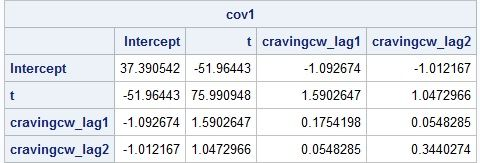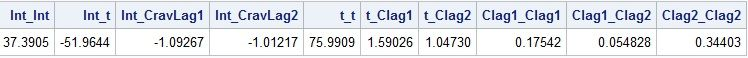## PROC IML: Need Help Moving Elements of a Matrix into a Vector of Columns, By Group

Hello All,

I have intensive longitudinal data with many observations per individual. I am running multiple regression models by individual and extracting both (1) parameter estimates and (2) the variance-covariance matrix for these estimates into a separate dataset.

I am looking to turn the variance-covariance matrix into a vector of columns, with each column containing just one of the matrix elements from the bottom half of the matrix (including the diagonal). I am able to achieve this for one individual using the following PROC IML code.

Here is the variance-covariance matrix of the estimates for individual 1 (what I start with).Here is the code I used:

``````proc iml;
use cov_id1; /*cov_id1 is the name of the dataset output from the proc reg ods output statement requesting covb */
read all var _num_ into cov1[r=Variable c=VarNames];
close cov_id1;
print cov1;
cov_vars1 = vech(cov1);
cov_vars_t1 = cov_vars1`;
print cov_vars_t1;
create cov_rows1
from cov_vars_t1[colname={"Int_Int" "Int_t" "Int_CravLag1" "Int_CravLag2"
"t_t" "t_Clag1" "t_Clag2" "Clag1_Clag1" "Clag1_Clag2" "Clag2_Clag2"}];
append from cov_vars_t1;
close cov_rows1;
quit; proc print data=cov_rows1 noobs; run;``````

Which produces this result for individual 1:HOWEVER, I am hitting a wall trying to get PROC IML to loop through each participant and output a row of matrix elements for each individual in the study (N = 200).

Does anyone have ideas for how to improve this code to allow me to create a dataset that has one row, marked with the ID, for each individual, and contains all of the unique variance-covariance matrix elements in separate columns for that individual?

I have attached a snippet of the data I am working with. As you can see, some of the elements will be missing for some individuals (see ID = 6), in case that complicates things.

Thank you.

4 REPLIES 4

## Re: PROC IML: Need Help Moving Elements of a Matrix into a Vector of Columns, By Group

Well, it will definitely be a problem if the model is changing between individuals. Notice that ID = 6 only has two observations. I assume the intercept is always present in the model. Any other tips about which effects are in the model? It makes the computations much harder.

The basic outline that you want to follow is

1. Read ID into one variable. Read the covariance columns into an N x 4 matrix. You might need to read "Variable", too.

3. Use EXPANDGRID to form the pairwise combinations of names. For example,

P = ExpandGrid(varNames, varNames);
PairNames = rowcatc(P[,1] + "_" + P[,2]);

4. Open a data set for writing and use the trick in (2) to loop over the unique ID values. For each ID, use row subscripts to get the covariance matrix.

5. You then need to extract the lower triangular elements. How you do this step depends on how the models vary. You could use VECH if all IDs had the same terms in the model.

I have a program that works when each ID has the same model, but I'll hold off posting until you report back.

## Re: PROC IML: Need Help Moving Elements of a Matrix into a Vector of Columns, By Group

Thank you -- to answer your question: yes, the intercept is always included in the model. The other effects are as follows:

t is a within-day time trend (linear)

cravingcw_lag1 is the immediately previous person-mean centered value of the DV (its effect is the first-order autoregression)

cravingcw_lag2 is the twice previous person-mean centered value of the DV (its effect is the second-order autoregression)

I've now attached the full data file I'm working with -- as you can see, the majority of observations have all 4 effects; a small number do not have enough observations in order to estimate the lagged effects, so these are missing for those individuals.Ksharp
Super User

## Re: PROC IML: Need Help Moving Elements of a Matrix into a Vector of Columns, By Group

``````proc corr data=sashelp.class out=temp cov noprint;
var age weight height;
run;

data have(drop=_TYPE_);
set temp;
if _TYPE_='COV';
run;
proc print;run;

proc iml;
use have ;
read all var _num_ into x[c=vname];
close;
idx=loc(row(x)>=col(x));
s=ndx2sub(nrow(x)||ncol(x),idx);
col=vname[s[,1]]+'_'+vname[s[,2]];
v=t(x[idx]);
create want from v[c=col];
append from v;
close;
quit;

proc print;run;``````

## Re: PROC IML: Need Help Moving Elements of a Matrix into a Vector of Columns, By Group

Have you made any progress on this by using the steps I outlined? Here is an IML program I wrote over the weekend, in case it is helpful. I hard-coded the check for the case where only two parameters (Intercept and t) are valid :

``````libname com 'put_your_ path_here';

proc iml;
start GetLowerTriang(A);
idx = loc(col(A) <= row(A));
return A[idx];
finish;

varNames = {"Intercept" "t" "Cravingcw_Lag1" "Cravingcw_Lag2"};
use com.cov_id; /*cov_id1 is the name of the dataset output from the proc reg ods output statement requesting covb */
read all var varNames into AllCov;
close;

/* https://blogs.sas.com/content/iml/2011/11/07/an-efficient-alternative-to-the-unique-loc-technique.html
*/
b = uniqueby(ID, 1);      /* b[i] = beginning of i_th category */
b = b // (nrow(ID)+1);     /* trick: append (n+1) to end of b */

P = ExpandGrid(varNames, varNames);
PairMat = shape( rowcatc(P[,1] + "_" + P[,2]), ncol(varNames));
AllPairNames = GetLowerTriang(PairMat);
print AllPairNames;
PairNames2 = GetLowerTriang(PairMat[1:2,]);
print PairNames2;

PairNames = AllPairNames;  /* initialize length */
create cov_rows var {"SubjID" "PairNames" "Cov"};;
do i = 1 to nrow(b)-1;    /* 4. For each level... */
idx = b[i]:(b[i+1]-1); /* 5. Find observations in level */
NN = ncol(idx);
C = AllCov[idx,];      /* the covariance matrix */
Cov = GetLowerTriang(C);   /* 6. Compute statistic on those values */
SubjID = repeat(ID[b[i]], nrow(Cov));
if NN = 2 then
PairNames = PairNames2;
else
PairNames = AllPairNames;
append;
end;
close cov_rows;
quit;``````
From The DO Loop